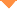首页 > 论文 > 激光与光电子学进展 > 57卷 > 9期(pp：91203--1)

A Pose Information Acquisition Method Based on Characteristic Line Intersection Analysis

• 摘要• 论文信息• 参考文献• 被引情况• PDF全文Abstract

To solve the problems of the lack of a model and inaccurate axis extraction of an airplane, a method for rendezvous analysis pose attainment of classified lines is proposed. In this method, first, a rotation matrix is obtained by deriving the unique correspondence between the pose definition and the progressive rotation of Euler angles in the reference coordinate system, and the lines are classified based on the carried pose information. Furthermore, a method for non-axis rendezvous analysis pose attainment, which uses the relative pose relationship between the feature line and the intersecting axis as input value for rotation transformation and obtains the rotation matrix by improving the feature line vector in the reference system, is implemented. Then, the pose rotation matrix is obtained by the modified Rodrigues transformation using the vector space relationship between the synchronous rotations of the two lines. The pose information can be analyzed using the solving time matrix. Tests show that the stability of the proposed algorithm is better than that of the model matching method, and its processing efficiency is considerably increased. Moreover, it has obvious advantages in terms of anti-occlusion, feature location, and tracking, which provides a new direction for the optical pose processing of the range.【2】Wang K P, Zhang X H, Yu Q F. Single station image attitude determination method based on the proportion of target characteristic points [J]. Applied Optics. 2009, 30(6): 974-978.

【5】Yu Q F, Sun X Y, Chen G J. A new method of measure the pitching and yaw of the axes symmetry object through the optical image [J]. Journal of National University of Defense Technology. 2000, 22(2): 15-19.

【6】Xi F, Wen G J, Cai W K. Estimating pose parameters of flying object by using model and stereo images [J]. Computer Simulation. 2007, 24(11): 95-99, 143.

【7】Su G Z. Research on the method of aircraft pose surveying from image sequences obtained by photoelectric theodolite [D]. Wuhan: Wuhan University. 2005.

Hu Xiaoli,Tang Minggang,Wu Haiying. A Pose Information Acquisition Method Based on Characteristic Line Intersection Analysis[J]. Laser & Optoelectronics Progress, 2020, 57(9): 091203